# Volume of Revolution Discussion (iv)

In this discussion, we are tasked with finding the rate of change of the volume function. By volume function, we refer for now to a function that maps a value on the positiveaxis to the volume of the solid formed when the function is rotated 360° around theaxis, between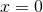and our chosen point.

With this function, the volume of the cylinder below, fromto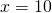is.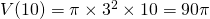.

Also, the volume fromtowould be..

Therefore the volume fromtois simplyThe volume of the cylinder in figure 1 is therefore.Figure 1.

Now let’s look at a more complicated curve. We are going to examine the section of the curve betweenand. Following the argument above, the volume of that brown solid is.Figure 2.

Let’s extract that rotated portion of the curve to see it more closely (Figure 3). We see that the function that forms it is increasing betweenand.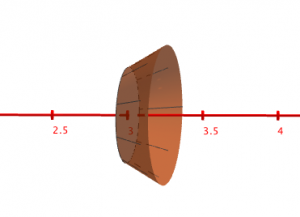Figure 3.

In Figure 4, we draw a cylinder that is smaller in volume that the actual solid we are studying. It is drawn with a radius that is the minimum value ofbetweenand, that is,. The length of the cylinder is 0.25. Using the familiar volume of cylinder formula, the volume of that cylinder is.Figure 4.

Now on figure 5 we see that a larger cylinder has been formed using the maximum value ofon the interval, which is. The volume of this cylinder is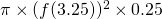.Figure 5.

The volume of the brown solid we are attempting to calculate lies between the volume of those two cylinders. We can write:Let’s generalise to two points reasonably close to each other. In place of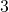lets useand in place oflet’s use– for now let’s suppose thatis positive. Let’s for the sake of argument suppose that our curve is increasing, thereforeis the minimum value ofon this interval and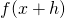is the maximum value ofon the interval.

Now, the volume of the brown solid can be written more generally as:Now our formula is beginning to look familiar. Let’s divide this whole expression by the positive number.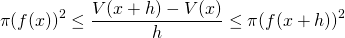Finally, let’s take the limit of each part of the expression astends to zero.

Left hand side:Right hand side: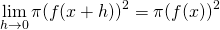Now it’s interesting, as the left and the right hand side of our inequalitytend to the same quantity. The expression in the middle must agree! Read about the pinching theorem (or squeeze theorem) here.

The middle:.

In other words, the rate of change of the volume function is equal to.

Now we haveit is a small step to claim thatandwhich takes care of the constant of integration. (In this case, simply the solution to V(0)=0).

Another way to think of this, perhaps intuitively, is to multiply the expressionby 1 so that it recognisably matches the formula for a simple cylinder. We recognise this expression to be that of the volume of a cylinder with length one and radius thevalue of the curve.

You may have noticed that our original simple example had a constant rate of change. In the example of the cylinder generated by the function, for every one unit increase on theaxis the volume increased by. So at any point on the more complicated curves, should the volume suddenly take on a constant rate of change, the curve would need to have the formconstant, and the solid would become at cylindrical at this point. As we know, we are not obliged to live out a full minute to get a one minute heart rate – we imagine the solid to become cylindrical just for an instant.